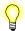# log1p(), log1pf(), log1pl()

Log(1+x)

## Synopsis:

```#include <math.h>

double log1p ( double x );

float log1pf ( float x );

long double log1pl( long double x );```

## Arguments:

x
The number that you want to add 1 to and compute the natural log of.

## Library:

libm

Use the -l m option to qcc to link against this library.

## Description:

The log1p(), log1pf(), and log1pl() functions compute the value of log(1 + x), where x > -1.0.

## Returns:

log(1 + x)

For finite values of x less than -1, these functions return NaN. The return value when x is -Inf is NaN.If an error occurs, these functions return 0, but this is also a valid mathematical result. If you want to check for errors, set errno to 0, call the function, and then check errno again. These functions don't change errno if no errors occurred.

## Classification:

Safety:
Cancellation point No
Interrupt handler No
Signal handler No